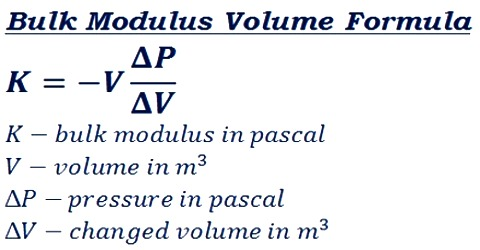# Significance Bulk Modulus

Significance Bulk modulus

Within elastic limit, the ratio of volume stress to volume strain is constant. This constant is called volume or bulk modulus. It is the ratio of the intensity of stress to the volume strain produced by stress —used of an elastic medium subjected to volume compression. Volume or bulk modulus of water is 2 x 109 Nm-2. This statement means that in order to create unit volume strain of water a force of 2 x 109 N is to be applied normally per unit area on all sides.

Compressibility: If equal pressure is applied on a body from all sides, then volume of the body decreases. This property of the body is called compressibility.

Since there are gaps between the molecules of a body, so compressibility occurs. Compressibility of a gas is much more compared to that in solid and liquid. Thus, the bulk modulus is a measure of resistance to compressibility of a fluid. A flat slope signifies a fairly compressible fluid having a low bulk modulus. A steep slope indicates a stiff, or only slightly compressible fluid

Mathematical definition: The reciprocal of bulk modulus is called compressibility.Sometimes bulk modulus is called incompressibility. Solids have all the elastic constants Y, K and n whereas liquids and gas have only bulk modulus.